• 初始化列表是什么
千次阅读
2021-05-07 21:42:36

初始化列表就是一种新型简单的有参构造函数，比以前的构造函数少写一点代码

代码一：

class Person {
public:

传统方式初始化
//Person(int a, int b, int c) {
//	m_A = a;
//	m_B = b;
//	m_C = c;
//}
//初始化列表方式初始化
Person() :m_A(10), m_B(20), m_C(30) {}
void PrintPerson() {
cout << "mA:" << m_A << endl;
cout << "mB:" << m_B << endl;
cout << "mC:" << m_C << endl;
}
private:
int m_A;
int m_B;
int m_C;
};
int main() {
Person p;
p.PrintPerson();
system("pause");
return 0;
}

代码二：

class Person {
public:
传统方式初始化
//Person(int a, int b, int c) {
//	m_A = a;
//	m_B = b;
//	m_C = c;
//}
//初始化列表方式初始化
Person(int a, int b, int c) :m_A(a), m_B(b), m_C(c) {}
void PrintPerson() {
cout << "mA:" << m_A << endl;
cout << "mB:" << m_B << endl;
cout << "mC:" << m_C << endl;
}
private:
int m_A;
int m_B;
int m_C;
};

int main() {
Person p(1, 2, 3);
p.PrintPerson();
system("pause");
return 0;
}

更多相关内容
• 主要介绍了C++11就地初始化列表初始化的相关资料，帮助大家更好的理解和学习C++,感兴趣的朋友可以了解下
• 1.只能在构造函数初始化列表初始化的成员变量的类型?  a.const成员变量  b.引用类型的成员变量  c.static不能在初始化列表中进行初始化  d.类成员变量中有自定义类型的变量最好在初始化列表中进行初始化 2.初始...
• ## c++中的初始化列表

千次阅读 2022-04-13 21:52:28
3.最好使用初始化列表进行初始化，因为对于自定义类型，我们还是需要使用初始化列表进行初始化的 4. 成员变量在类中声明次序就是其在初始化列表中的初始化顺序，与其在初始化列表中的先后次序无关 初始化列表的...

目录

初始化列表的概念和使用

初始化列表的注意事项

1. 每个成员变量在初始化列表中只能出现一次(初始化只能初始化一次)

2. 类中包含以下成员，必须放在初始化列表位置进行初始化：

3.最好使用初始化列表进行初始化，因为对于自定义类型，我们还是需要使用初始化列表进行初始化的

4. 成员变量在类中声明次序就是其在初始化列表中的初始化顺序，与其在初始化列表中的先后次序无关

## 初始化列表的概念和使用

初始化列表：以一个冒号开始，接着是一个以逗号分隔的数据成员列表，每个"成员变量"后面跟一个放在括号中的初始值或表达式

Data(int year = 0, int month = 0, int day = 0) : _year(year), _month(month), _day(day)
//以构造函数声明后的:(冒号)开始，每个成员变量用,(逗号)隔开，()括号中放的是初始化的值
{}

## 初始化列表的注意事项

一个对象的当个成员变量在初始化列表是定义阶段(构造函数)，而在构造函数里面就是赋值了，假如我们没写成员变量的初始化列表也会初始化，只不过初始化为随机值

### 2. 类中包含以下成员，必须放在初始化列表位置进行初始化：

1、引用成员变量

class Data
{
public:
Data(int year = 0, int month = 0, int day = 0):_a(year)	//使用初始化列表初始化
{
_year = year;
_month = month;
_day = day;
}

private:
int _year;
int _month;
int _day;
int& _a;	//定义一个引用成员变量
};

引用在类中只是被声明，没有被定义，所以可以先不初始化，当我们实例化一个对象之后，必须进行初始化，所以引用要在初始化列表初始化

2、const成员变量

class Data
{
public:
Data(int year = 0, int month = 0, int day = 0):_a(0)	//使用初始化列表初始化
{
_year = year;
_month = month;
_day = day;
}

private:
int _year;
int _month;
int _day;
const int _a;	//定义一个const修饰的成员变量
};

const修饰的成员变量不能够被修改，所以需要在定义时候进行初始化

3、自定义类型成员(该类没有默认构造函数）

class A
{
public:
A(int x)	//因为我们写了构造函数，所以这个类没有默认构造函数了
{
_a = x;
}
public:
int _a;
};

class Data
{
public:
Data(int year = 0, int month = 0, int day = 0) :a(0)	//使用初始化列表初始化
{
_year = year;
_month = month;
_day = day;
}

private:
int _year;
int _month;
int _day;
A a;	//创建一个类的对象
};

因为类没有默认构造函数，所以类里面的成员变量不会自动赋值，我们必须对它进行初始化，这个初始化就在初始化列表

### 4. 成员变量在类中声明次序就是其在初始化列表中的初始化顺序，与其在初始化列表中的先后次序无关

#include<iostream>
using namespace std;

class A
{
public:
A(int a)
:_a1(a)
, _a2(_a1)    //使用初始化列表初始化
{}
void Print() {
cout << _a1 << " " << _a2 << endl;
}
private:
int _a2;
int _a1;
};

int main() {
A aa(1);
aa.Print();
}

结果为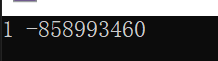因为初始化列表进行初始化的时候是按照成员变量声明的顺序来的，所以_a2先被初始化为随机值，_a1再被初始化为a

展开全文c语言 c++
• ## 详解初始化列表

千次阅读 2017-08-08 13:01:04
初始化列表定义构造函数有个特殊的初始化方式叫“初始化表达式表”（简称初始化列表）。初始化列表位于函数参数表之后，却在函数体 {} 之前。这说明该表里的初始化工作发生在函数体内的任何代码被执行之前。Date(int...

## 初始化列表

### 定义

构造函数有个特殊的初始化方式叫“初始化表达式表”（简称初始化列表）。初始化列表位于函数参数表之后，却在函数体 {} 之前。这说明该表里的初始化工作发生在函数体内的任何代码被执行之前。

Date(int year, int month, int day)//带参构造函数
:_year(year)
, _month(month)
, _day(day)
{}

### 构造函数的两个执行阶段

构造函数的执行可以分成两个阶段，初始化阶段和计算阶段，初始化阶段先于计算阶段。

#### 初始化阶段：

所有类类型（class type）的成员都会在初始化阶段初始化，即使该成员没有出现在构造函数的初始化列表中。

#### 计算阶段

一般用于执行构造函数体内的赋值操作。

### 为什么使用初始化列表

使用初始化列表主要是基于性能问题，对于内置类型，如int, float等，使用初始化类表和在构造函数体内初始化差别不是很大，但是对于类类型来说，最好使用初始化列表，为什么呢？我们看例子：

使用构造函数函数体内赋值：

class Test1
{
public:
Test1()//无参的构造函数
{
cout << "Construct Test1" << endl;
}
Test1(const Test1& t1) // 拷贝构造函数
{
cout << "Copy constructor for Test1" << endl;
}

Test1& operator=(const Test1& t1)
{
cout << "operator=()" << endl;
a = t1.a;
return *this;
}
protected:
int a;
};

class Test2
{
public:
Test1 test1;

Test2(Test1 &t1)
{
test1 = t1;
}
};
int main()
{
Test1 t1;
Test2 t2(t1);
system("pause");
return 0;
}


输出结果：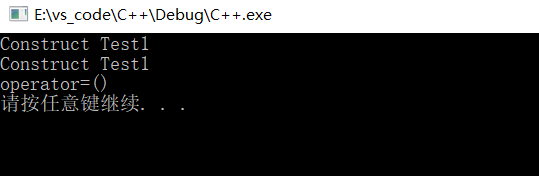使用初始化列表赋值：

class Test1
{
public:
Test1()//无参的构造函数
{
cout << "Construct Test1" << endl;
}
Test1(const Test1& t1) // 拷贝构造函数
{
cout << "Copy constructor for Test1" << endl;
}

Test1& operator=(const Test1& t1)
{
cout << "operator=()" << endl;
a = t1.a;
return *this;
}
protected:
int a;
};

class Test2
{
public:
Test1 test1;

Test2(Test1 &t1)
:test1(t1)
{}
};
int main()
{
Test1 t1;
Test2 t2(t1);
system("pause");
return 0;
}

输出结果：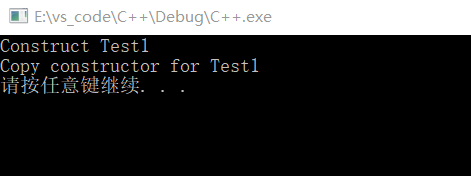从上面的两个代码比较可以看出使用初始化列表，直接调用拷贝构造函数初始化，省去了默认构造函数的过程。所以能使用初始化列表的时候尽量使用初始化列表。

### 哪些东西必须放在初始化列表中

• 常量成员，因为常量只能初始化不能赋值，所以必须放在初始化列表里面。
• 引用类型，引用必须在定义的时候初始化，并且不能重新赋值，所以也要写在初始化列表里面。
• 没有默认构造函数的类类型，因为使用初始化列表可以不必调用默认构造函数来初始化，而是直接调用拷贝构造函数初始化。

### 成员变量的初始化顺序

成员是按照他们在类中声明的顺序进行初始化的，而不是按照他们在初始化列表出现的顺序初始化的。例如：

class Date
{
public:
Date(int year, int month, int day)//带参构造函数
:_month(month)//第二个初始化
, _year(year) //第一个初始化
, _day(day)   //第三个初始化
{}

private:
int _year; //第一个定义
int _month;//第二个定义
int _day;  //第三个定义
};
展开全文c++ 构造函数
• 主要介绍了C++ 初始化列表详解及实例代码的相关资料,需要的朋友可以参考下
• ## 【C++】-- 初始化列表

千次阅读 多人点赞 2022-04-19 22:36:18
初始化列表：以一个冒号开始，接着是一个以逗号分隔的数据成员列表，每个"成员变量"后面跟一个放在括 号中的初始值或表达式。

目录

一、用初始化列表初始化对象

1.初始化列表用法

2.初始化列表特性

二、explicit关键字

1.内置类型的隐式转换

2.如何避免单参构造函数初始化发生隐式类型转换创建一个类对象时，编译器通过调用构造函数，给类对象中各个成员变量赋初值：

class Date
{
public:
//构造函数
Date(int year = 2022, int month = 4, int day = 19)
{
_year = year;
_month = month;
_day = day;
}

private:
int _year;
int _month;
int _day;
};


但上述赋初值不能称作类对象成员的初始化，因为构造函数体内可以多次赋值：

class Date
{
public:
//构造函数
Date(int year = 2022, int month = 4, int day = 19)
{
_year = year;
_month = month;
_day = day;
_year = 2023;//构造函数体内允许对成员变量进行多次赋值
}

private:
int _year;
int _month;
int _day;
};


而初始化列表能只能初始化一次。

# 一、用初始化列表初始化对象

## 1.初始化列表用法

初始化列表：以一个冒号开始，接着是一个以逗号分隔的数据成员列表，每个"成员变量"后面跟一个放在括 号中的初始值或表达式。

class Date
{
public:
//构造函数
Date(int year = 2022, int month = 4, int day = 19)
:_year(year) //初始化列表初始化
,_month(month)
,_day(day)
{}

private:
int _year;
int _month;
int _day;
};


## 2.初始化列表特性

（1）初始化列表能只能初始化一次，多次初始化会报错：

class Date
{
public:
//构造函数
Date(int year = 2022, int month = 4, int day = 19)
:_year(year)
,_month(month)
,_day(day)
,_month(month) //初始化列表多次初始化
{}

private:
int _year;
int _month;
int _day;
};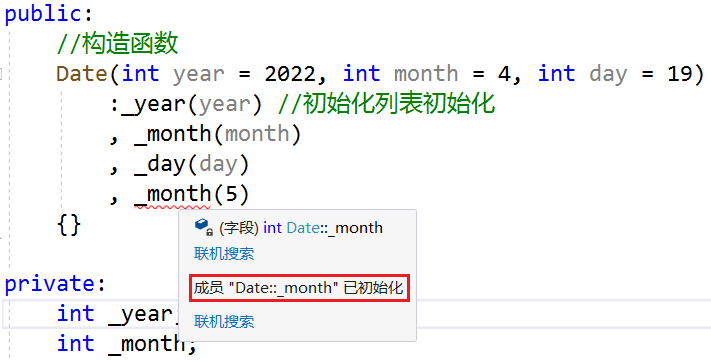编译器也允许构造函数赋初值和初始化列表初始化混用：

class Date
{
public:
//构造函数
Date(int year = 2022, int month = 4, int day = 19)
:_year(year)  //两者混用
,_month(month)
{
_day = day;
}

private:
int _year;
int _month;
int _day;
};


混用时初始化列表初始化和构造函数赋初值不冲突：

class Date
{
public:
//构造函数
Date(int year = 2022, int month = 4, int day = 19)
: _year(year) //两者不冲突
, _month(month)
{
_day = day;
_year = 2023;
}

private:
int _year;
int _month;
int _day;
};


但混用时初始化列表初始化还是要遵循只能初始化一次成员变量的原则：

class Date
{
public:
//构造函数
Date(int year = 2022, int month = 4, int day = 19)
: _year(year) //初始化列表初始化
, _month(month)
, _year(2023)  //_year在初始化列表里被初始化了两次，不允许
{
_day = day;
}

private:
int _year;
int _month;
int _day;
};


（2）const成员变量、引用成员变量、没有默认构造函数的自定义类型成员只能在初始化列表初始化。

①const成员变量必须在定义的时候初始化

class Date
{
public:
//构造函数
Date(int year = 2022, int month = 4, int day = 19)
: _year(year)
, _month(month)
, _n(2) //const成员变量必须使用初始化列表进行初始化
{
_day = day;
//_n = 2; //const成员变量不能在函数体内初始化
}

private:
int _year;
int _month;
int _day;

const int _n = 1;
};

②引用成员变量必须在定义的时候初始化

class Date
{
public:
//构造函数
Date(int year = 2022, int month = 4, int day = 19)
: _year(year)
, _month(month)
,_ref(year)//引用成员变量要在初始化列表初始化
{
_day = day;
//_ref = year; //引用成员变量不能在函数体内初始化
}

private:
int _year;
int _month;
int _day;

int& _ref;
};


③没有默认构造函数的自定义类型成员变量

#include <iostream>
using namespace std;

class A
{
public:

//默认构造函数是不用传参就可以调用的构造函数，有3种：
//1.无参默认构造函数
//2.带参全缺省的默认构造函数
//3.我们不写，编译器自动生成的默认构造函数

A(int x)//不属于以上任何一种，所以A类的对象没有默认构造函数
{
cout << "A(int x)" << endl;
_x = x;
}

private:
int _x;
};

class Date
{
public:
//构造函数
Date(int year = 2022, int month = 4, int day = 19)
: _year(year)
, _month(month)
, _a(20)//没有默认构造函数的自定义类型成员变量必须在初始化列表进行初始化
{
_day = day;
}

private:
int _year;
int _month;
int _day;

A _a;
};


const成员变量、引用成员变量、没有默认构造函数的自定义类型成员变量必须在初始化列表内初始化的原因：

①初始化列表是对象的成员变量定义的地方。

②对象的内置类型成员变量在初始化列表定义时没有要求必须初始化，因此既可以在初始化列表进行初始化，也可以在构造函数体内初始化。

③而const成员变量、引用成员变量、没有默认构造函数的自定义类型成员变量不能先定义再初始化，它们在初始化列表内定义，并且必须在定义时就初始化，因此必须在初始化列表内初始化。

（3） 尽量使用初始化列表初始化，因为不管是否使用初始化列表，虽然对于内置类型没有差别，但是对于自定义类型成员变量，一定会先使用初始化列表初始化。

为什么会先使用初始化列表初始化？

如下，Date类没有默认构造函数，因为26行的构造函数不属于默认构造函数中的任意一种，在对Date类的对象d进行初始化时，会调用Date类的默认构造函数，所以对象d的day实参12和hour实参12都没有被传进去，_t作为Date类的自定义类型成员变量会调用Time类的默认构造函数，_hour默认传参为0，因此打印_hour的值也为0，d的参数没有传成功：

#include<iostream>
using namespace std;

class Date; // 前置声明
class Time
{
friend class Date; // 声明日期类为时间类的友元类，则在日期类中就直接访问Time类中的私有成员变量
public:
Time(int hour = 0)
: _hour(hour)
{
cout << _hour << endl;
}

private:
int _hour;
};

class Date
{
public:
Date(int day, int hour)
{}

private:
int _day;
Time _t;
};

int main()
{
Date d(12, 12);
return 0;
}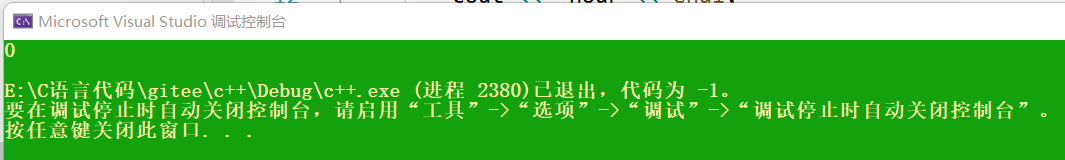假如Date类的构造函数不使用初始化列表进行初始化，使用函数体内初始化时，要把Date类的构造函数的形参hour的值给d，那么就必须构造一个Time类对象t，对该对象传参传hour，再使用赋值运算符重载函数将对象t拷贝给_t：

#include<iostream>
using namespace std;

class Date; // 前置声明
class Time
{
friend class Date; // 声明日期类为时间类的友元类，则在日期类中就直接访问Time类中的私有成员变量
public:
Time(int hour = 0)
: _hour(hour)
{
cout << _hour << endl;
}

private:
int _hour;
};

class Date
{
public:
//自定义类型，不使用初始化列表，就需要使用构造函数 + operator=
Date(int day, int hour)
{
//函数体内初始化
Time t(hour);//调用Time类的构造函数
_t = t;

_day = day;
}

private:
int _day;
Time _t;
};

int main()
{
Date d(12, 12);
cout << 4 << endl;
return 0;
}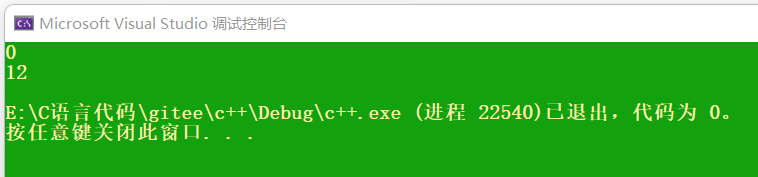这还不如直接使用使用初始化列表初始化呢，还不需要赋值运算符重载函数：

class Date
{
public:
//自定义类型，使用初始化列表，只需要构造函数
Date(int day, int hour)
:_t(hour)
{
_day = day;
}

private:
int _day;
Time _t;
};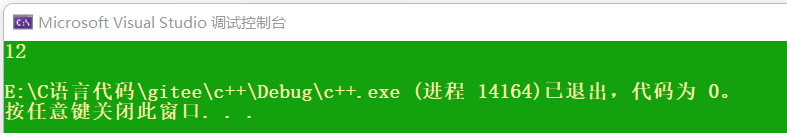因此，建议尽量直接使用初始化列表进行初始化。

（4）成员变量初始化的顺序就是成员变量在类中的声明次序，与初始化列表中的先后次序无关。

如下代码，类成员变量中先声明了_a2，再声明了_a1，因此初始化的顺序是先初始化_a2，再初始化_a1：

#include <iostream>
using namespace std;
class A
{
public:
A(int a)
: _a1(a)
, _a2(_a1)
{}

void Print()
{
cout << _a1 << " " << _a2 << endl;
}
private:
int _a2;//先声明_a2
int _a1;//后声明_a1
};

int main() {
A aa(1);
aa.Print();
}

先声明_a2就会先初始化_a2，用_a1初始化_a2，由于此时_a1还是随机值，因此_a2的值也是随机值，_a1使用a的值1进行初始化，因此，_a1的值为1：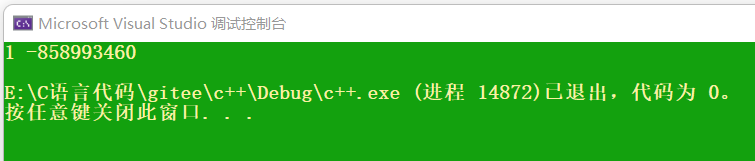所以，建议类中的成员变量声明的顺序和初始化列表中初始化的顺序一致。

# 二、explicit关键字

## 1.内置类型的隐式转换

int i = 0;
double d = i;//隐式类型转换

根据监视可以看出：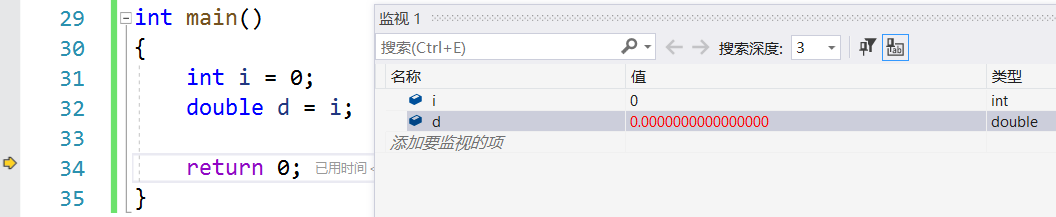double d = i;并不是将i直接赋值给d，而是用i创建一个临时变量，再把临时变量的值给d，那么d改变的是临时变量的值，而不是i的值，因为程序执行完毕后，i的值并未发生改变。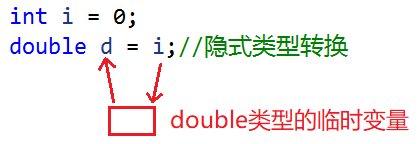如果d作为引用，那么必须加上const关键字进行修饰，因为d不是i的引用，是临时变量的引用，而临时变量具有常性，不允许引用权限放大。

int i = 0;
const double& d = i;//d引用了临时变量，临时变量具有常性，所以d也必须具有常性

## 2.如何避免单参构造函数初始化发生隐式类型转换

正常的类对象初始化如下面的aa1，也可以使用拷贝构造初始化，如aa2。由于c++支持隐式类型转换，因此也支持单参数构造函数初始化，如aa3：

#include<iostream>
using namespace std;

class A
{
public :
A(int a)
:_a(a)
{}

private:
int _a;
};

int main()
{
A aa1(1);//构造aa1对象
A aa2(aa1);//拷贝构造，程序没写拷贝构造，编译器会自动生成拷贝构造函数，对内置类型完成浅拷贝

A aa3 = 3;//单参数的构造函数，会发生隐式类型转换

return 0;
}

那么

A aa3 = 3;

是如何支持类型转换的呢？

对于自定义类型A，aa3是A类型，3是整形。编译器会先拿A构造一个临时对象temp，3作为参数传给这个临时对象temp，再拿aa3(temp)去拷贝构造，发生隐式类型转换，即先构造，再拷贝构造：

//A aa3 = 3;
A temp(3);   //先构造
A aa3(temp); //再拷贝构造

不过现在的编译器已经优化过了，会直接调用构造函数A aa(3)。

如果不想让单参数的构造函数发生隐式类型转换，可以使用explicit关键字修饰构造函数，表明该构造函数是显式的，而不是隐式的，就会避免发生不期望的类型转换，使用场景如下：

#include<iostream>
using namespace std;

class A
{
public:
A(int a)
:_a(a)
{}

private:
int _a;
};

int main()
{
A aa1(1);//构造aa1对象
A aa2(aa1);//拷贝构造，程序没写拷贝构造，编译器会自动生成拷贝构造函数，对内置类型完成浅拷贝

A aa3 = 'x';//先拿A构造一个临时对象temp，字符x作为参数传给这个临时对象temp，会发生隐式类型转换，再拿aa3(temp)去拷贝构造

return 0;
}

aa3作为A类的对象，构造时传参应该传int型，但却传了char型，由于发生隐式类型转换，因此编译也没毛病，但是它传参就是不伦不类。这时候可以给A的构造函数加上explicit声明不让该单参构造函数发生隐式类型转换，编译就会报错：

class A
{
public:
explicit A(int a)
:_a(a)
{}

private:
int _a;
};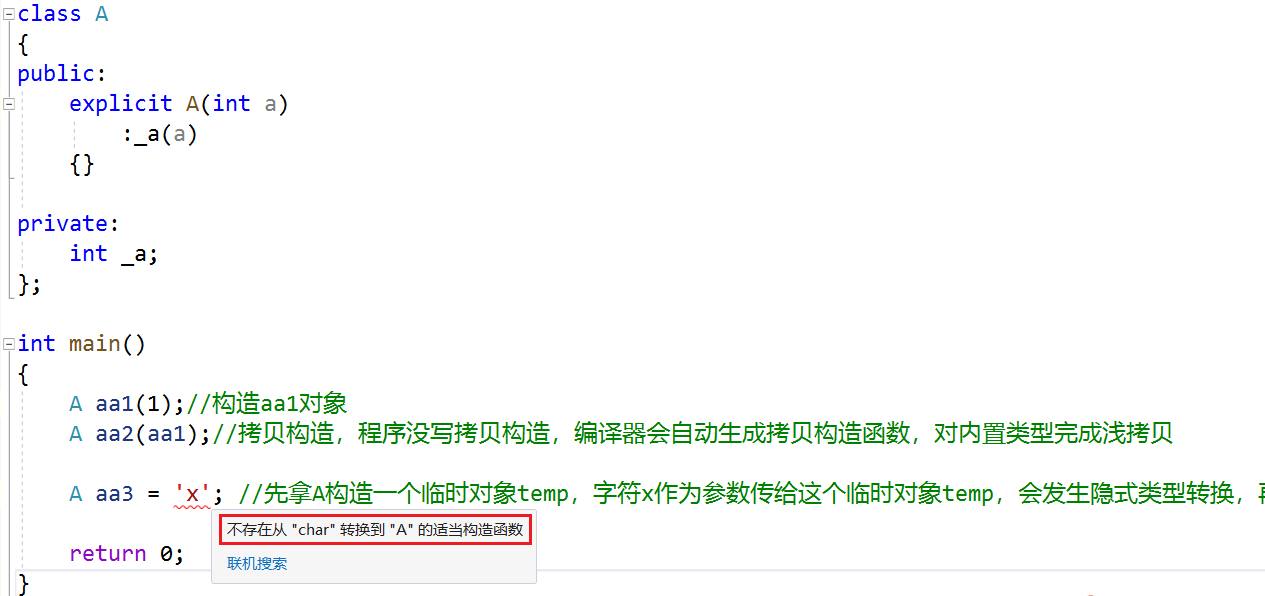这时候只能乖乖给aa3传int型参数了。

# 三、匿名对象

## 1.匿名对象定义

没有名字的对象叫做匿名对象，A(3)跟aa1和aa2相比少了个对象名，没有名字，aa1和aa2的生命周期在main函数内，A(3)的生命周期只在当前行：

#include<iostream>
using namespace std;

class A
{
public:
explicit A(int a)
:_a(a)
{
cout << "A(int a):"<< a << endl;
}

A(const A& aa)
{
cout << "A(const A&)" << endl;
}

~A()
{
cout << "~A()" << endl;
}

private:
int _a;
};

int main()
{
A aa1(1);//生命周期在main函数内
A aa2(aa1);//生命周期在main函数内

A(3);//构造匿名对象，生命周期只在这一行

return 0;
}

F10调试：当执行完A(3)还没执行return 0时，aa1和aa2的生命周期还没有结束，不会调用析构函数，此时打印的析构函数只能是匿名对象A(3)的析构函数：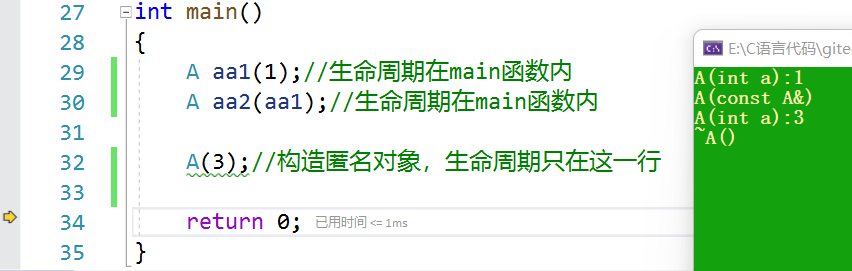所以A(3)这一行执行完就调析构函数了。

## 2.匿名对象应用场景

假设有一个函数f，且A类的构造函数全缺省：

#include<iostream>
using namespace std;

class A
{
public:
A(int a = 0)//构造函数全缺省
:_a(a)
{
cout << "A(int a):"<< a << endl;
}

A(const A& aa)
{
cout << "A(const A&)" << endl;
}

~A()
{
cout << "~A()" << endl;
}

void f()//f函数
{
cout << "f()" << endl;
}

private:
int _a;
};

int main()
{
A aa1(1);//生命周期在main函数内
A aa2(aa1);//生命周期在main函数内

A(3);//构造匿名对象，生命周期只在这一行

return 0;
}

调用f()函数时，需要定义一个A类对象，才能调用A类函数f，这就需要写两行：

int main()
{
A aa1(1);//生命周期在main函数内
A aa2(aa1);//生命周期在main函数内

A(3);//构造匿名对象，生命周期只在这一行

A aa4;//需要定义一个A类对象，才能调用f
aa4.f();

return 0;
}

对象aa4 在main函数结束后才会销毁。如果定义对象只是为了调用函数，那么可以考虑直接定义一个匿名对象：

int main()
{
A aa1(1);//生命周期在main函数内
A aa2(aa1);//生命周期在main函数内

A(3);//构造匿名对象，生命周期只在这一行

A aa4;//需要定义一个A类对象，才能调用f
aa4.f();

A().f();//定义匿名对象来调用函数f()

return 0;
}

这个匿名对象就是为了调用函数f，这个匿名对象后边也没人用它，在当前行调用完f()函数就销毁了。

展开全文开发语言
• 什么时候必须使用初始化列表 1. 初始化 != 赋值. a.初始化代表为变量分配内存. 变量在其定义处被编译器初始化(编译时). 在函数中, 函数参数初始化发生在函数调用时(运行时). b.赋值代表"擦除对象当前值, 赋予新值". ...c++
• ## Python初始化列表

万次阅读 多人点赞 2019-04-29 18:17:49
Python初始化列表 下面我们就来介绍在Python中初始化列表（我们创建大小为1000并使用零初始化的列表）的一些方法。 1）方法一：使用for循环和append()函数 arr = [] for i in range(1000): arr.append(0) 2）方法...Python
• ## C++ 初始化列表

千次阅读 2021-03-04 16:24:03
那我们怎样才能完成成员变量的初始化呢，这时候就引进了–初始化列表 格式： 以一个冒号开始，接着是一个以逗号分隔的数据成员列表，每个"成员变量"后面跟一个放在括号中的初始值或表达式 class Date { public: ...c++
• Python是一种非常灵活的语言，可以通过多种方式执行单个任务，例如，可以通过多种方式执行初始化列表。下面本篇文章就来带大家了解几种Python初始化的方法，并比较一下这几种方法的效率，希望对大家有所帮助。下面...
• ## c++中的初始化列表详解

万次阅读 多人点赞 2018-05-18 18:15:04
与其他函数不同，构造函数除了有名字，参数列表和函数体之外，还可以有初始化列表初始化列表以冒号开头，后跟一系列以逗号分隔的初始化字段。 class foo { public: foo(string s, int i):name(s), id(i){} ; //...c++ 构造函数
• 什么是初始化列表，来看下面代码，我们有一个日期类对象 我们可以这样来定义构造函数： class Date ...
• 一、什么是初始化列表？ 以一个冒号开始，接着是一个以逗号分隔的数据成员列表，每个"成员变量"后面跟一个放在括号中的初始值或表达式。 class Date { public: Date(int year, int month, int day) : _year...c++
• ## C++初始化列表详解

千次阅读 2020-07-01 12:21:32
使用初始化列表的原因         必须使用初始化列表的时候         成员变量的顺序 定义 与其他函数不同，构造函数除了有名字，...c++
•c++
• 类的构造函数的作用是对其成员进行初始化。而在构造函数中可以使用初始值列表为新创建的对象的成员进行赋值。 1 初始值列表 在类的构造函数名之后紧跟着冒号，冒号后面是要初始化的成员名，之后是圆括号或者花括号...C++ C++11 构造函数
• 详细介绍列表初始化适用于哪些场景以及列表初始化所具有的优点，每个观点均有详细的代码验证，方便理解！c++
• 本文探讨C++构造函数体内初始化与列表初始化的区别： ...2、若类B通过初始化列表去初始化，则只调用类A的拷贝构造函数。 另外，虽然对于成员类型是内置类型的情况，通过上述两种情况去初始化是相同的，但是为了标...C++
• 一般的初始化是使用小括号()。 给数组初始化是使用大括号{}。 class base{ char a; public: base():a{{}}{} } 上例的初始化就相当于：a = {};。
• 使用初始化列表有两个原因： 原因1.必须这样做：《C++ Primer》中提到在以下三种情况下需要使用初始化成员列表： 情况一、需要初始化的数据成员是对象的情况(这里包含了继承情况下，通过显示调用父类的构造函数对...c++
• 学习了一下前缀树。 需要用到一个结构体保存两个指针，如下 struct Node ...能不能将初始化操作放在初始化列表中呢，如下 以上两种写法都有问题，突然想到，数组应该用大括号初始化啊 就酱。 ...
• 初始化列表1. 类成员为const类型2 . 类成员为引用类型3.没有默认构造函数的类类型4. 如果类存在继承关系，派生类必须在其初始化列表中调用基类的构造函数 1. 类成员为const类型 2 . 类成员为引用类型 1.常量成员，...
• 当定义结构体变量时，可以通过两种方式初始化它：使用初始化列表或构造函数。编程语言 c++
• c++类的初始化列表，定义，使用场景，使用方法分析c++
• } 初始化列表与默认参数配合使用 如果函数声明和实现是分离的，初始化列表只能写在函数的实现中，默认参数只能写在函数的声明中。 #include using namespace std; struct Person { int m_age; int m_height; ...c++
• 引用类型的成员变量必须在构造函数的初始化列表中进行初始化。对于类成员是const修饰，或是引用类型的情况，是不允许赋值操作的，(显然嘛，const就是防止被错误赋值的，引用类型必须定义赋值在......开发语言
• ## 成员初始化列表

千次阅读 2017-03-18 17:44:29
2、成员初始经列表由逗号分隔的初始化列表组成(前面带冒号)。它位于参数列表的右括号之后、函数体左括号之前。 3、初值可以是常量或构造函数的参数列表中的参数。这种方法并不限于初始化常量。 例如下代码： Queue:......